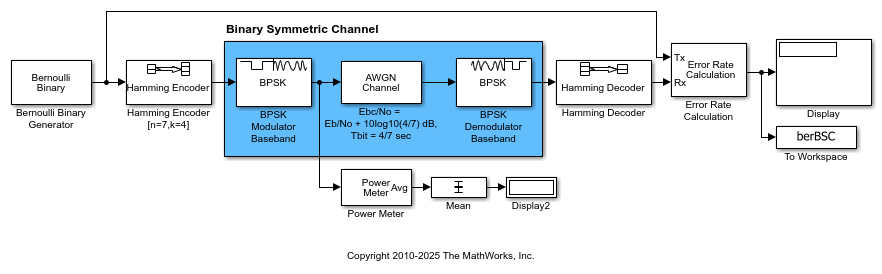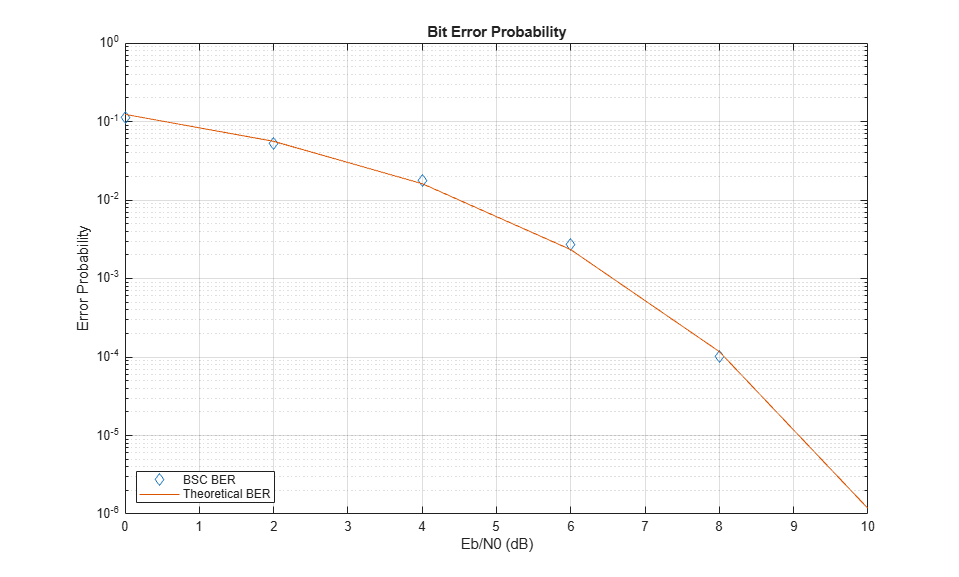# Using AWGN Channel Block for Coded Signals

When your simulation uses coding, the Eb/N0 setting for the AWGN channel must be adjusted by the coding rate to apply the desired signal to noise (SNR) ratio.

The `slex_hamming_check.slx` model performs forward error control (FEC) coding on a BPSK-modulated signal that gets filtered through an AWGN channel. The model uses BPSK Modulator Baseband, AWGN Channel, and BPSK Demodulator Baseband discrete blocks to simulate a binary symmetric channel. To account for difference between the coded and uncoded Eb/N0 (Eb/N0), the AWGN channel block computes the coded Eb/N0 as Eb/N0 + 10log10(K/N) dB, where K/N is the code rate and Eb/N0 is the uncoded Eb/N0. The Hamming Encoder block has an input bit period of 1 second and the output bit period decreases, by a factor of the K/N code rate, to 4/7 seconds. Due to the coding rate, the Binary Symmetric Channel has a bit period of 4/7 seconds.

The model initializes variables used to configure block parameters by using the `PreLoadFcn` callback function. For more information, see Model Callbacks (Simulink).

```model = 'slex_hamming_check'; open_system(model); ```The AWGN channel block must also configure the Number of bits per symbol and Input signal power, referenced to 1 ohm (watts) parameter settings based on the modulated signal. This example uses BPSK modulation, so the AWGN Channel block has number of bits per symbol set to `1`. The model includes a Power Meter block that measures the signal power at the AWGN input to confirm the setting required for the input signal power of the AWGN Channel block.

Produce error rate results and a plot to compare simulation with theory. The theoretical channel error probability for the coded signal is Q(sqrt(2*Ebc/N0)), where Q() is the standard Q function and Ebc/N0 is the coded Eb/N0 in linear units (not in dB). Compute the theoretical BER upper limit bound of a linear, rate 4/7 block code with a minimum distance of 3, and hard decision decoding for a BPSK-modulated signal in AWGN over a range of Eb/N0 values by using the `bercoding` function. Simulate the `slex_hamming_check` model over the same range of Eb/N0 values.

```EbNoVec = 0:2:10; theorBER = bercoding(EbNoVec,'block','hard',7,4,3); berVecBSC = zeros(length(EbNoVec),3); for n = 1:length(EbNoVec) EbNo = EbNoVec(n); sim(model); berVecBSC(n,:) = berBSC(end,1); end ```

Plot the results by using the `semilogy` function to show the nearly identical results. The model has the Error Rate Calculation block configured to run each Eb/N0 point until 200 errors occur or the block receives 1x10^6 bits.

```semilogy( ... EbNoVec,berVecBSC(:,1),'d', ... EbNoVec,theorBER,'-'); legend('BSC BER','Theoretical BER', ... Location="southwest"); xlabel("Eb/N0 (dB)"); ylabel("Error Probability"); title("Bit Error Probability"); grid on; ```